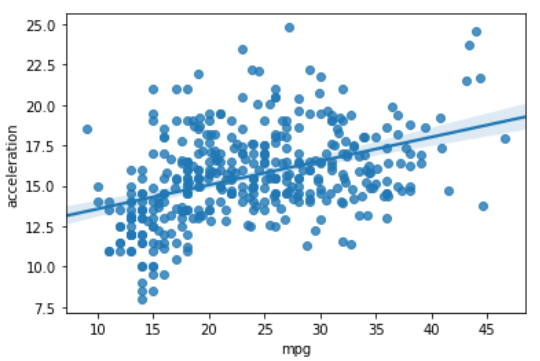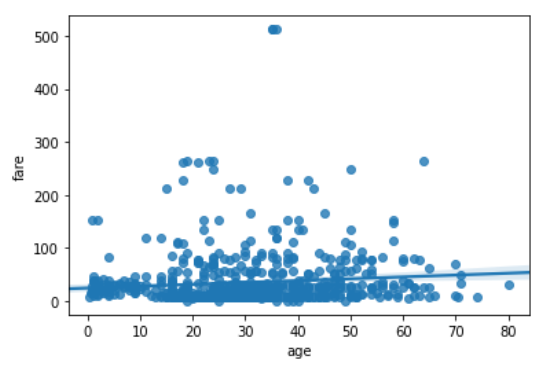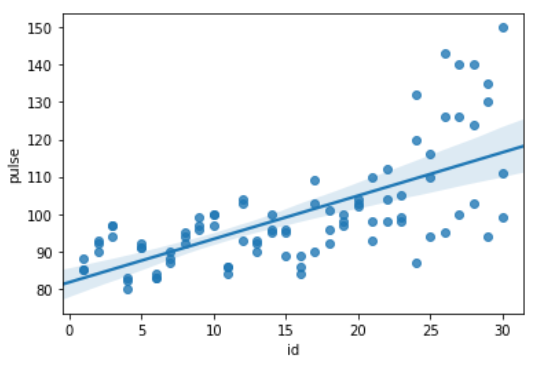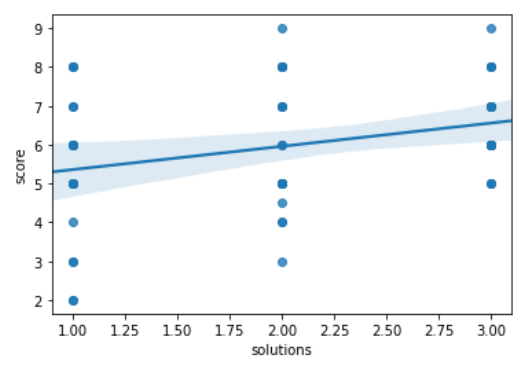Open In App

# Python – seaborn.regplot() method

Seaborn is a Python data visualization library based on matplotlib. It provides a high-level interface for drawing attractive and informative statistical graphics. Seaborn helps resolve the two major problems faced by Matplotlib; the problems are ?

• Default Matplotlib parameters
• Working with data frames

As Seaborn compliments and extends Matplotlib, the learning curve is quite gradual. If you know Matplotlib, you are already half-way through Seaborn.

### seaborn.regplot() :

This method is used to plot data and a linear regression model fit. There are a number of mutually exclusive options for estimating the regression model. For more information click here.

Syntax : seaborn.regplot( x,  y,  data=None, x_estimator=None, x_bins=None,  x_ci=’ci’, scatter=True, fit_reg=True, ci=95, n_boot=1000, units=None, order=1, logistic=False, lowess=False, robust=False, logx=False, x_partial=None, y_partial=None, truncate=False, dropna=True, x_jitter=None, y_jitter=None, label=None, color=None, marker=’o’,    scatter_kws=None, line_kws=None, ax=None)

Parameters: The description of some main parameters are given below:

• x, y: These are Input variables. If strings, these should correspond with column names in “data”. When pandas objects are used, axes will be labeled with the series name.
• data:  This is dataframe where each column is a variable and each row is an observation.
• lowess: (optional) This parameter take boolean value. If “True”, use “statsmodels” to estimate a nonparametric lowess model (locally weighted linear regression).
• color: (optional) Color to apply to all plot elements.
• marker: (optional) Marker to use for the scatterplot glyphs.

Return: The Axes object containing the plot.

Below is the implementation of above method:

Example 1:

## Python3

 `# importing required packages``import` `seaborn as sns``import` `matplotlib.pyplot as plt` `# loading dataset``data ``=` `sns.load_dataset(``"mpg"``)` `# draw regplot``sns.regplot(x ``=` `"mpg"``,``            ``y ``=` `"acceleration"``,``            ``data ``=` `data)` `# show the plot``plt.show()` `# This code is contributed``# by Deepanshu Rustagi.`

Output :Example 2:

## Python3

 `# importing required packages``import` `seaborn as sns``import` `matplotlib.pyplot as plt` `# loading dataset``data ``=` `sns.load_dataset(``"titanic"``)` `# draw regplot``sns.regplot(x ``=` `"age"``,``            ``y ``=` `"fare"``,``            ``data ``=` `data,``            ``dropna ``=` `True``)``# show the plot``plt.show()` `# This code is contributed``# by Deepanshu Rustagi.`

Output :Example 3:

## Python3

 `# importing required packages``import` `seaborn as sns``import` `matplotlib.pyplot as plt` `# loading dataset``data ``=` `sns.load_dataset(``"exercise"``)` `# draw regplot``sns.regplot(x ``=` `"id"``,``            ``y ``=` `"pulse"``,``            ``data ``=` `data)` `# show the plot``plt.show()` `# This code is contributed``# by Deepanshu Rustagi.`

Output :Example 4 :

## Python3

 `# importing required packages``import` `seaborn as sns``import` `matplotlib.pyplot as plt` `# loading dataset``data ``=` `sns.load_dataset(``"attention"``)` `# draw regplot``sns.regplot(x ``=` `"solutions"``,``            ``y ``=` `"score"``,``            ``data ``=` `data)` `# show there plot``plt.show()` `# This code is contributed``# by Deepanshu Rustagi.`

Output :